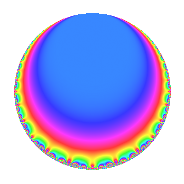# Properties

 Label 1800.2.a.iLevel 1800 Weight 2 Character orbit 1800.a Self dual Yes Analytic conductor 14.373 Analytic rank 1 Dimension 1 CM No Inner twists 1

# Related objects

## Newspace parameters

 Level: $$N$$ = $$1800 = 2^{3} \cdot 3^{2} \cdot 5^{2}$$ Weight: $$k$$ = $$2$$ Character orbit: $$[\chi]$$ = 1800.a (trivial)

## Newform invariants

 Self dual: Yes Analytic conductor: $$14.3730723638$$ Analytic rank: $$1$$ Dimension: $$1$$ Coefficient field: $$\mathbb{Q}$$ Coefficient ring: $$\mathbb{Z}$$ Coefficient ring index: $$1$$ Fricke sign: $$1$$ Sato-Tate group: $\mathrm{SU}(2)$

## $q$-expansion

 $$f(q)$$ $$=$$ $$q - 2q^{7} + O(q^{10})$$ $$q - 2q^{7} + 2q^{11} - 4q^{13} + 2q^{17} + 4q^{19} - 8q^{23} - 10q^{29} + 4q^{31} + 8q^{43} - 8q^{47} - 3q^{49} - 6q^{53} - 14q^{59} - 14q^{61} + 4q^{67} + 12q^{71} - 6q^{73} - 4q^{77} - 12q^{79} - 4q^{83} - 12q^{89} + 8q^{91} + 14q^{97} + O(q^{100})$$

## Embeddings

For each embedding $$\iota_m$$ of the coefficient field, the values $$\iota_m(a_n)$$ are shown below.

For more information on an embedded modular form you can click on its label.

Label $$\iota_m(\nu)$$ $$a_{2}$$ $$a_{3}$$ $$a_{4}$$ $$a_{5}$$ $$a_{6}$$ $$a_{7}$$ $$a_{8}$$ $$a_{9}$$ $$a_{10}$$
1.1
 0
0 0 0 0 0 −2.00000 0 0 0
 $$n$$: e.g. 2-40 or 990-1000 Significant digits: Format: Complex embeddings Normalized embeddings Satake parameters Satake angles

## Inner twists

This newform does not admit any (nontrivial) inner twists.

## Atkin-Lehner signs

$$p$$ Sign
$$2$$ $$1$$
$$3$$ $$1$$
$$5$$ $$1$$

## Hecke kernels

This newform can be constructed as the intersection of the kernels of the following linear operators acting on $$S_{2}^{\mathrm{new}}(\Gamma_0(1800))$$:

 $$T_{7} + 2$$ $$T_{11} - 2$$ $$T_{13} + 4$$